# Electronics and Communication Engineering - Automatic Control Systems

### Exercise :: Automatic Control Systems - Section 2

21.

For the second order system having following differential equation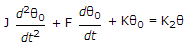(When θ0 and θi are output and input) the natural frequency is

 A.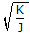B.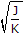C. KJ D.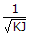Explanation:

Compare with standard form.

22.

For a second order system, the position of poles is in the shaded region shown in the given figure. The variation in damping factor is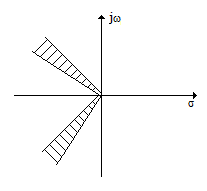A. from 0 to 1 B. from about 0.3 to 0.7 C. from 1 to 5 D. from 0 to 3

Explanation:

Variation in damping factor is small.

23.

For a factor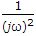in the transfer function, the log magnitude plot

 A. is a straight line with slope 0 dB/decade B. is a straight line with slope 20 dB/decade C. is a straight line with slope - 20 dB/decade D. is a straight line with slope - 40 dB/decade

Explanation:

jω term in denominator gives a straight line with a slope of - 20 dB/decade and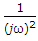gives a straight line with a slope of - 40 dB/decade.

24.

The frequency at which magnitude of closed loop frequency response is 3 dB below its zero frequency value is called critical frequency.

 A. True B. False

Explanation:

It is not critical frequency.

25.

The transfer function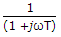has the characteristics of high pass filter.

 A. True B. False